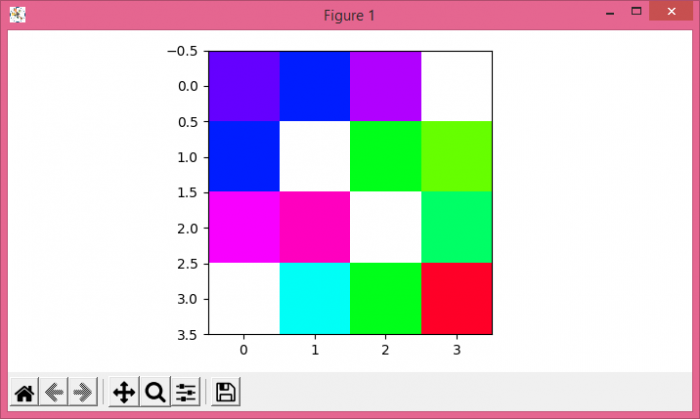# How to plot and work with NaN values in Matplotlib?

To plot and work with NaN values in matplotlib, we can take the following steps −

• Create data using numpy with some NaN values.

• Use imshow() method to display data as an image, i.e., on a 2D regular raster, with a colormap and data (from step 1).

• To display the figure, use show() method.

## Example

import numpy as np
from matplotlib import pyplot as plt
plt.rcParams["figure.figsize"] = [7.00, 3.50]
plt.rcParams["figure.autolayout"] = True
data = np.array([[1., 1.2, 0.89, np.NAN],
[1.2, np.NAN, 1.89, 2.09],
[.78, .67, np.NAN, 1.78],
[np.NAN, 1.56, 1.89, 2.78]]
)
plt.imshow(data, cmap="gist_rainbow_r")
plt.show()

## Output